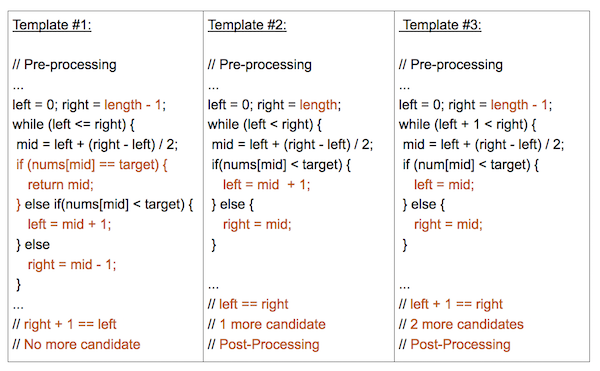# Leetcodes Binary Search Template II

Leetcodes的官方BS教程, 说分析了上百个案例,总结出三个BS模板 (需要注册才能看到链接)

3 Parts of a Successful Binary Search

Binary Search is generally composed of 3 main sections:

1. Pre-processing - Sort if collection is unsorted.
2. Binary Search - Using a loop or recursion to divide search space in half after each comparison.
3. Post-processing - Determine viable candidates in the remaining space.

Template #2 is an advanced form of Binary Search. It is used to search for an element or condition which requires accessing the current index and its immediate right neighbor’s index in the array.

``````def binarySearch(nums, target):
"""
:type nums: List[int]
:type target: int
:rtype: int
"""
if len(nums) == 0:
return -1

left, right = 0, len(nums)
while left < right:
mid = (left + right) // 2
if nums[mid] == target:
return mid
elif nums[mid] < target:
left = mid + 1
else:
right = mid

# Post-processing:
# End Condition: left == right
if left != len(nums) and nums[left] == target:
return left
return -1
``````

Key Attributes:

• An advanced way to implement Binary Search.
• Search Condition needs to access element’s immediate right neighbor
• Use element’s right neighbor to determine if condition is met and decide whether to go left or right
• Gurantees Search Space is at least 2 in size at each step
• Post-processing required. Loop/Recursion ends when you have 1 element left. Need to assess if the remaining element meets the condition.Leetcodes有模有样的用范式的三段论, 最后的的Post-processing 是画蛇添足.

``````def binarySearch(nums, target):
"""
:type nums: List[int]
:type target: int
:rtype: int
"""
if len(nums) == 0:
return -1

left, right = 0, len(nums)
while left < right:
mid = (left + right) // 2
if nums[mid] == target:
return mid
elif nums[mid] < target:
left = mid + 1
else:
right = mid

# Post-processing:
# End Condition: left == right
# if left != len(nums) and nums[left] == target:
#  return left
# 全部去掉, 一样能完美工作.
return -1
``````

post processing 是说要按需处理，比如找到了返回第一个还是最后一个，没找到返回 false，-1 还是 插入位置之类的。